# CAT 2015 – Paper Analysis

## Overview

• The overall structure of CAT-2015 was very different from that of CAT 2014.
• Each section was timed for 60 minutes. One could not go back and forth between sections.
• The test started with the Verbal Section, which had 34 questions – 24 RC and 10 VA.
• The second section was the DI-LR with 32 questions distributed equally between the two areas.
• The third section was the Quantitative Ability section which also had 34 questions.
• For the first time CAT introduced the TITA question type; these were open-ended questions where test-takers would have to type in the correct answer.
• As expected, non-MCQ questions did not carry any negative marks while the MCQ questions carried -0.33 mark negative for a wrong answer and +1 for a correct answer as displayed in actual test and +3 and -1 as shared in the instructions sheet before the start of the exam.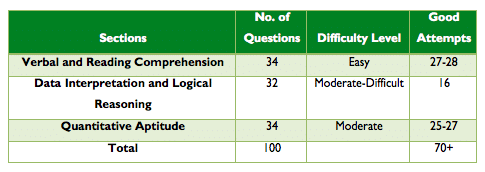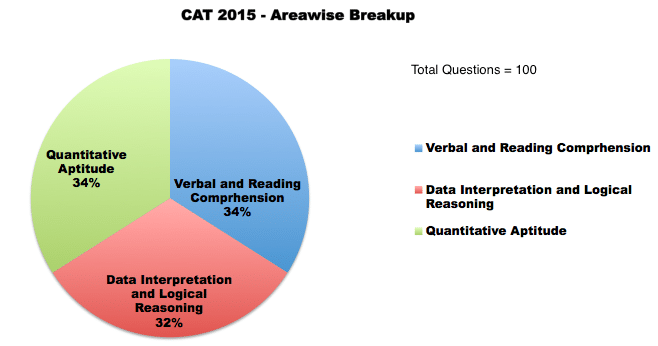### Verbal Ability and Reading Comprehension

• The passages were easy to understand and no passage had very abstract or dense subject matter
• There were total 5 passages – three passages (450-650 words in length) and two passages (300 words each). The three passages had 6 questions each and the other two had 3 questions each.
• Two parajumble questions were difficult and two were easy.
• There were no vocabulary-based questions as well.
• The questions in Verbal ability were dominated by Verbal Logic and did not carry any negative marking, though they needed prior practice to aim for a high percentile.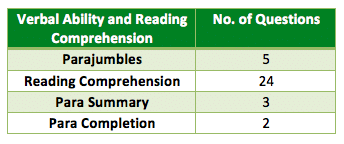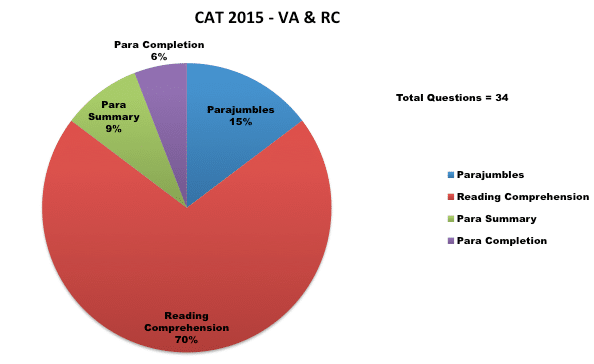### Data Interpretation and Logical Reasoning

• The DI-LR section had 4 sets each of Data Interpretation and Logical Reasoning.
• In DI three sets were easy and the one based on set theory was of moderate-difficult level
• Selection of the right sets to solve was key to a good performance in this section.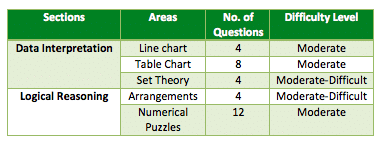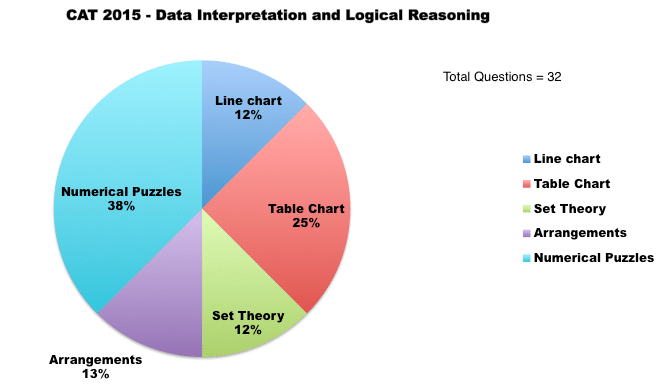### Quantitative Ability

• This section was of moderate difficulty level and dominated by questions on Algebra, Geometry and Arithmetic.
• There were 34 questions of QA. There were 15 Questions of Non MCQ type and the rest were MCQs.
• There were questions from regular topics like Algebra, Geometry, Modern Math, Arithmetic and Number Systems.
• The difficulty level of QA is marginally higher than CAT 2014.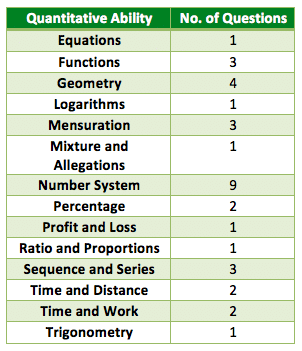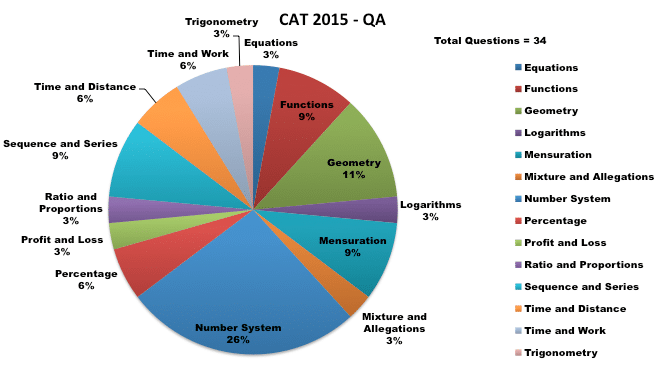### Verdict

CAT 2015 was pretty much on the lines of what they have already given on the CAT 2015 website. Students reported that there was a minor glitch in the Instructions given at the start of the paper. The Instructions said that there would be DILR questions in sets of 2 or 4 and Reading Comprehension (RC) questions in sets of 4.

With QA becoming slightly easier and more importantly, reduction in weightage for higher maths and the addition of an online calculator is a bit of help for non engineers, which had helped to increase diversity this year.